# Multiple Input Production Economics for Farm Management AAE

• Slides: 55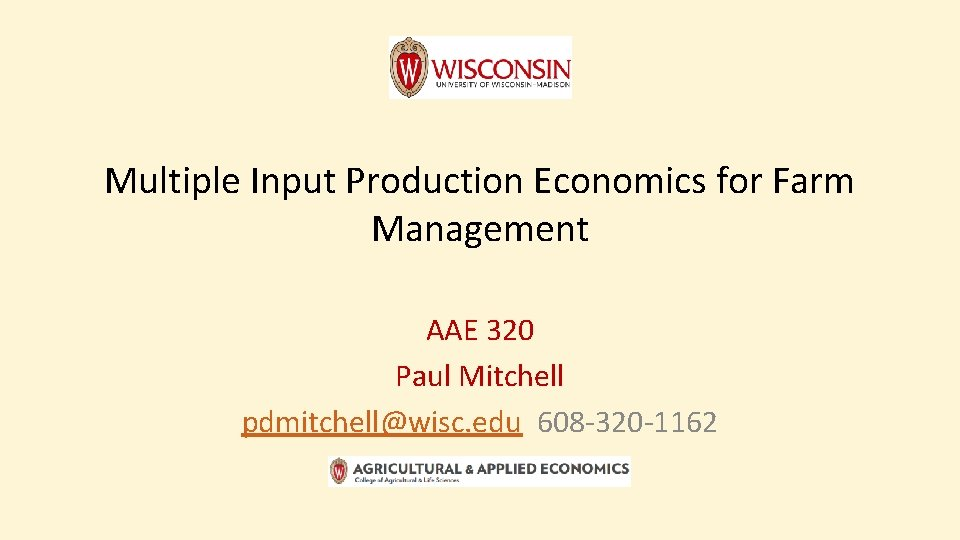Multiple Input Production Economics for Farm Management AAE 320 Paul Mitchell [email protected] edu 608 -320 -1162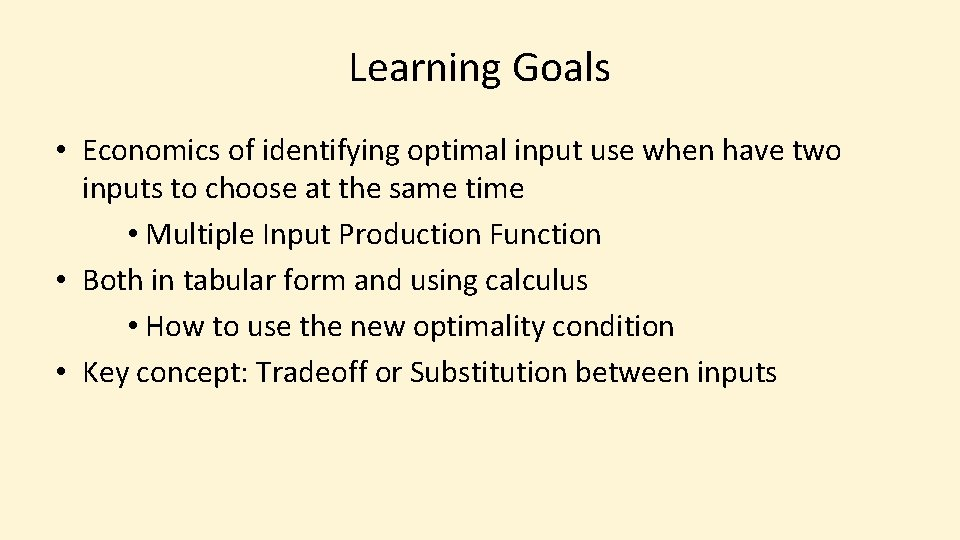Learning Goals • Economics of identifying optimal input use when have two inputs to choose at the same time • Multiple Input Production Function • Both in tabular form and using calculus • How to use the new optimality condition • Key concept: Tradeoff or Substitution between inputs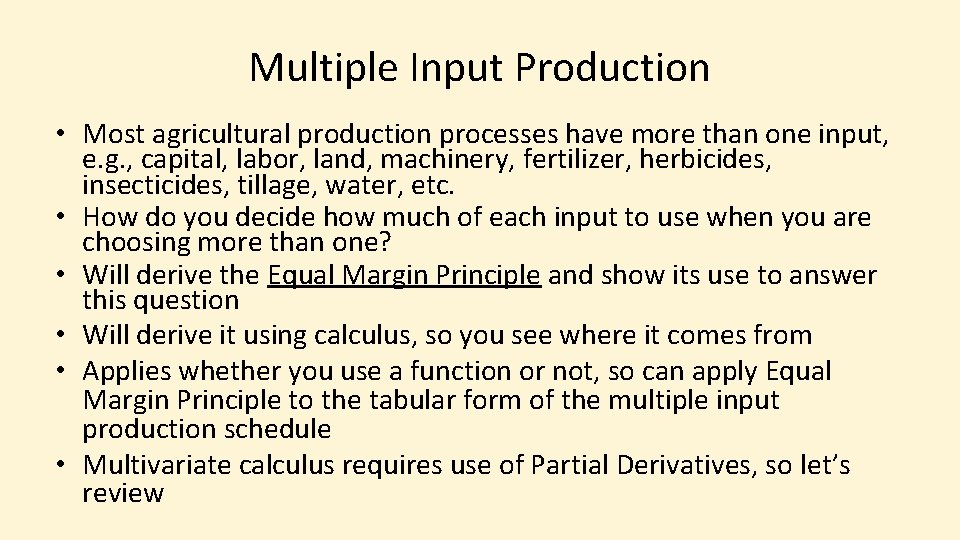Multiple Input Production • Most agricultural production processes have more than one input, e. g. , capital, labor, land, machinery, fertilizer, herbicides, insecticides, tillage, water, etc. • How do you decide how much of each input to use when you are choosing more than one? • Will derive the Equal Margin Principle and show its use to answer this question • Will derive it using calculus, so you see where it comes from • Applies whether you use a function or not, so can apply Equal Margin Principle to the tabular form of the multiple input production schedule • Multivariate calculus requires use of Partial Derivatives, so let’s reviewPartial Derivatives • Derivative of function that has more than one variable • What’s the derivative of Q = f(x, y)? Depends on which variable you are talking about. • Remember a derivative is the slope • Derivative of Q = f(x, y) with respect to x is the slope of the function in the x direction • Derivative of Q = f(x, y) with respect to y is the slope of the function in the y directionPartial Derivatives • Think of a hill; its elevation Q is a function of the location in latitude (x) and in longitude (y): Q = f(x, y) • At any spot on the hill, defined by a latitude-longitude pair (x, y), the hill will have a slope in the x direction and in the y direction Q • Slope in x direction: d. Q/dx = fx(x, y) Y • Slope in y direction: d. Q/dy = fy(x, y) XPartial Derivatives • Notation: if have Q = f(x, y) • First Partial Derivatives § d. Q/dx = fx(x, y) and d. Q/dy = fy(x, y) • Second (Own) Partial Derivative § d 2 Q/dx 2 = fxx(x, y) and d 2 Q/dy 2 = fyy(x, y) • Second Cross Partial Derivative § d 2 Q/dxdy = fxy(x, y)Partial Derivatives • Partial derivatives are the same as regular derivatives, just treat the other variables as constants • Q = f(x, y) = 2 + 3 x + 6 y – 2 x 2 – 3 y 2 – 5 xy • When taking the derivative with respect to x, treat y as a constant and vice versa • Q = f(x, y) = 2 + 3 x + 6 y – 2 x 2 – 3 y 2 – 5 xy • fx(x, y) = 3 – 4 x – 5 y • Q = f(x, y) = 2 + 3 x + 6 y – 2 x 2 – 3 y 2 – 5 xy • fy(x, y) = 6 – 6 y – 5 xThink Break #6 • Give the 1 st and 2 nd derivatives [fx(x, y), fy(x, y), fxx(x, y), fyy(x, y), fxy(x, y)] of each function: • f(x, y) = 7 + 5 x + 2 y – 5 x 2 – 4 y 2 – 11 xy • f(x, y) = – 5 – 2 x + y – x 2 – 3 y 2 + 2 xyEqual Margin Principle • Given production function Q = f(x, y), find (x, y) to maximize p(x, y) = pf(x, y) – rxx – ryy – K • FOC’s: dp/dx = 0 and dp/dy = 0 and solve for pair (x, y) • dp/dx = pfx(x, y) – rx = 0 • dp/dy = pfy(x, y) – ry = 0 • Just p x MPx = rx and p x MPy = ry Same as before • Just MPx = rx/p and MPy = ry/p • These still hold, but we have moreEqual Margin Principle • • Profit Maximization again implies p x MPx = rx and p x MPy = ry Note p x MPx = rx depends on y and p x MPy = ry depends on x These are two equations and both must be satisfied, so rearrange by making the ratio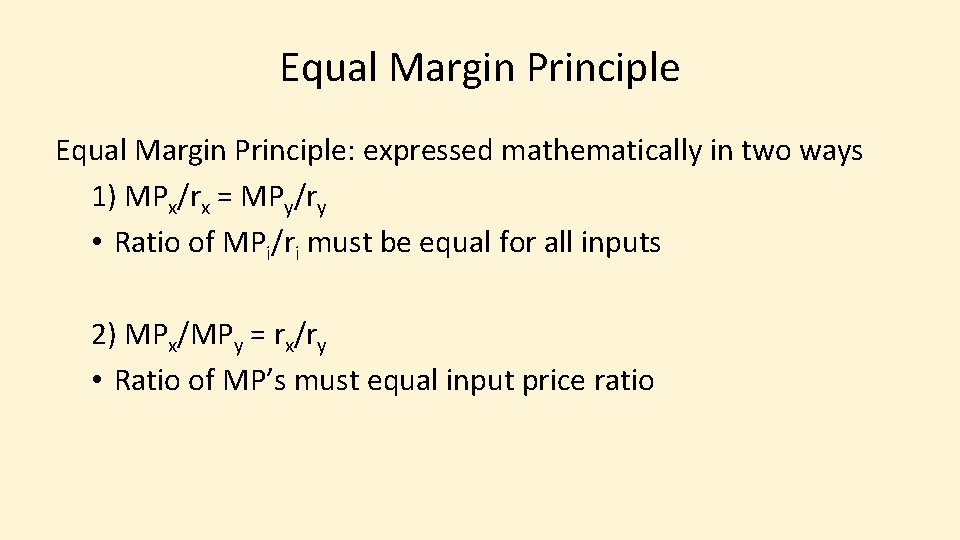Equal Margin Principle: expressed mathematically in two ways 1) MPx/rx = MPy/ry • Ratio of MPi/ri must be equal for all inputs 2) MPx/MPy = rx/ry • Ratio of MP’s must equal input price ratioIntuition for MPx/rx = MPy/ry Corn Example MPi is bu of corn from the last lb of N fertilizer (bu/lb) ri is \$ per lb of N fertilizer (\$/lb) Units for MPi/ri (bu/lb) / (\$/lb) = bu/\$ MPi/ri is bu of corn from the last \$ spent on N fertilizer MPi/ri is how many bushels of corn you get from the last dollar spent on N fertilizer • MPx/rx = MPy/ry means use each input so that the last dollar spent on each input gives the same extra output • • •Intuition for MPx/MPy = rx/ry Corn Example • MPx is bu of corn from last lb of N fertilizer (bu/lb N) • MPy is bu of corn from last 1 k seeds planted (bu/1 k seeds) • MPx/MPy = (1 k seeds/lbs N) is how many seeds/ac need to increase if cut N by 1 lb/ac and want to keep yield the same • Ratio of marginal products is the substitution rate between Nitrogen and Seeds in the production process • Have not talked about substitution between inputs, but the Ratio of the marginal products is the slope of the tradeoff curve between inputsIntuition for MPx/MPy = rx/ry Corn Example • rx is \$ per lb of N fertilizer (\$/lb N) • ry is \$ per 1 k seeds (\$/1 k seeds) • rx/ry is (\$/lb N)/(\$/1 k seeds) = 1 k seeds/lbs N, or the substitution rate between Nitrogen and Seeds in the market • MPx/MPy = rx/ry means use inputs so that the substitution rate between inputs in the production process is the same as the substitution rate between inputs in the market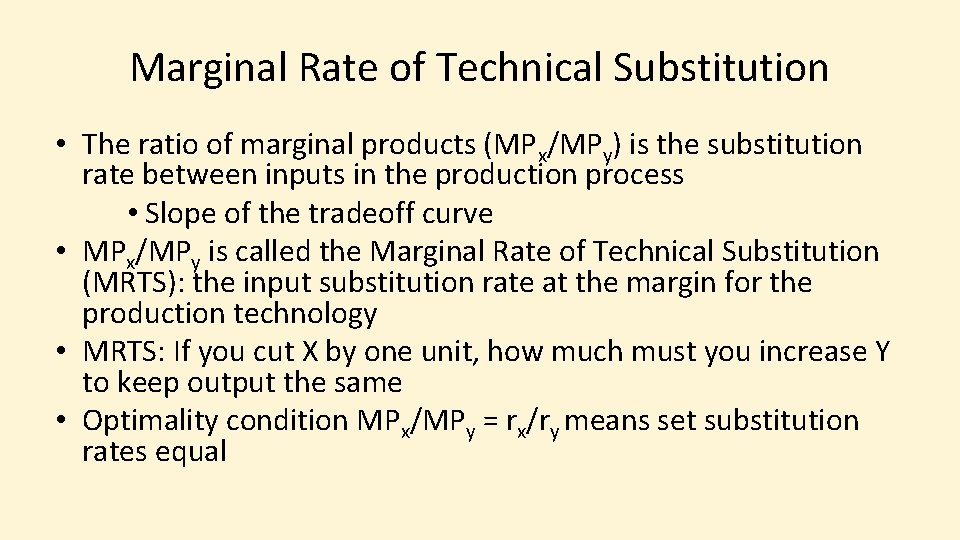Marginal Rate of Technical Substitution • The ratio of marginal products (MPx/MPy) is the substitution rate between inputs in the production process • Slope of the tradeoff curve • MPx/MPy is called the Marginal Rate of Technical Substitution (MRTS): the input substitution rate at the margin for the production technology • MRTS: If you cut X by one unit, how much must you increase Y to keep output the same • Optimality condition MPx/MPy = rx/ry means set substitution rates equalEqual Margin Principle Intuition • MPx/rx = MPy/ry means use inputs so the last dollar spent on each input gives the same extra output at the margin § Compare to p x MP = r or VMP = r • MPx/MPy = rx/ry means use inputs so the substitution rate at the margin between inputs is the same in the production process as in the marketplace § Compare to MP = r/p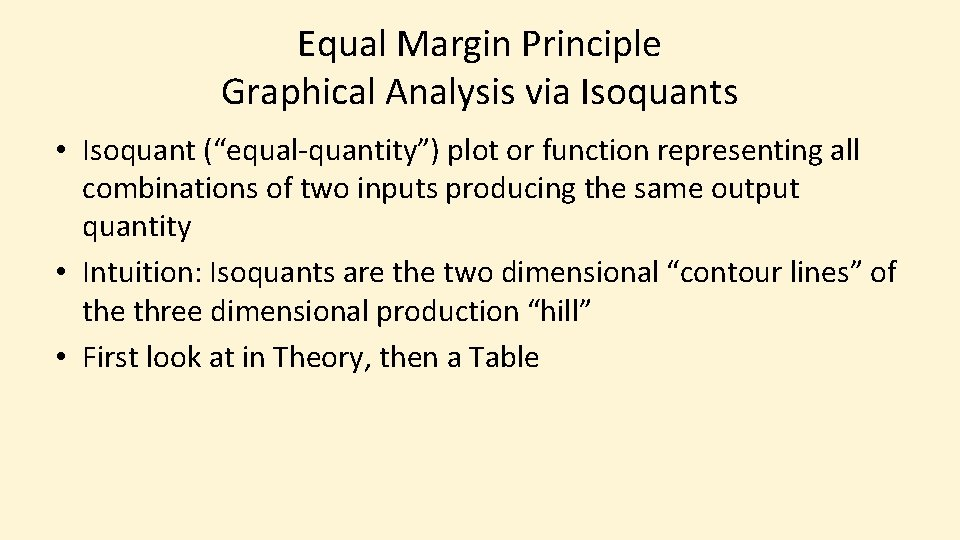Equal Margin Principle Graphical Analysis via Isoquants • Isoquant (“equal-quantity”) plot or function representing all combinations of two inputs producing the same output quantity • Intuition: Isoquants are the two dimensional “contour lines” of the three dimensional production “hill” • First look at in Theory, then a TableInput Y Isoquants in Theory ~ Output Q = Q Input X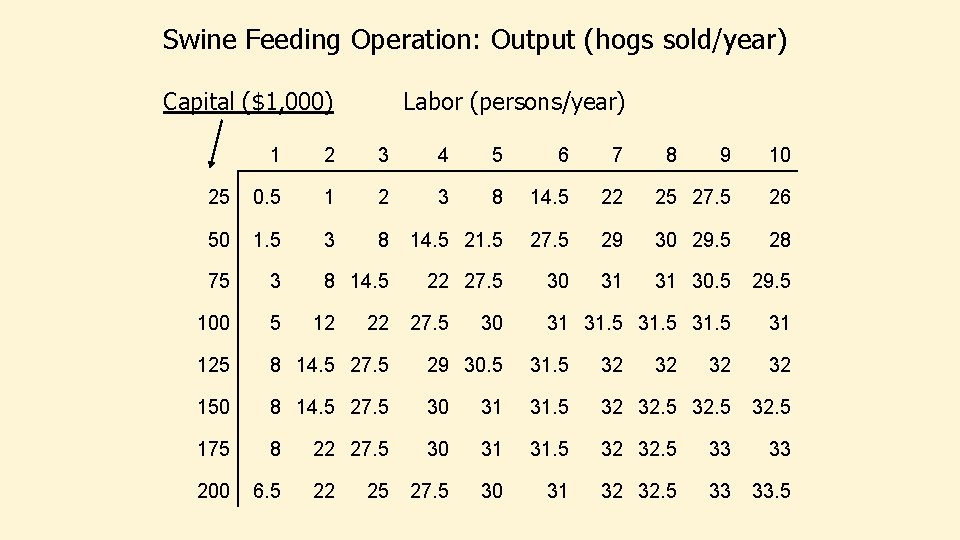Swine Feeding Operation: Output (hogs sold/year) Capital (\$1, 000) Labor (persons/year) 1 2 3 4 5 6 7 25 0. 5 1 2 3 8 14. 5 50 1. 5 3 8 14. 5 21. 5 75 3 8 14. 5 22 27. 5 100 5 125 8 14. 5 27. 5 29 30. 5 31. 5 32 32 32 150 8 14. 5 27. 5 30 31 31. 5 32 32. 5 175 8 30 31 31. 5 32 32. 5 33 33 200 6. 5 27. 5 30 31 32 32. 5 33 33. 5 12 22 22 27. 5 22 25 27. 5 30 8 9 10 22 25 27. 5 26 27. 5 29 30 29. 5 28 30 31 31 30. 5 29. 5 31 31. 5 31 32Swine feeding operation: Output (hogs sold/year) Capital (\$1, 000) Labor (persons/year) 1 2 3 4 5 6 7 25 0. 5 1 2 3 8 14. 5 50 1. 5 3 8 14. 5 21. 5 75 3 8 14. 5 22 27. 5 100 5 125 8 14. 5 27. 5 29 30. 5 31. 5 32 32 32 150 8 14. 5 27. 5 30 31 31. 5 32 32. 5 175 8 30 31 31. 5 32 32. 5 33 33 200 6. 5 27. 5 30 31 32 32. 5 33 33. 5 12 22 22 27. 5 22 25 27. 5 30 8 9 10 22 25 27. 5 26 27. 5 29 30 29. 5 28 30 31 31 30. 5 29. 5 31 31. 5 31 32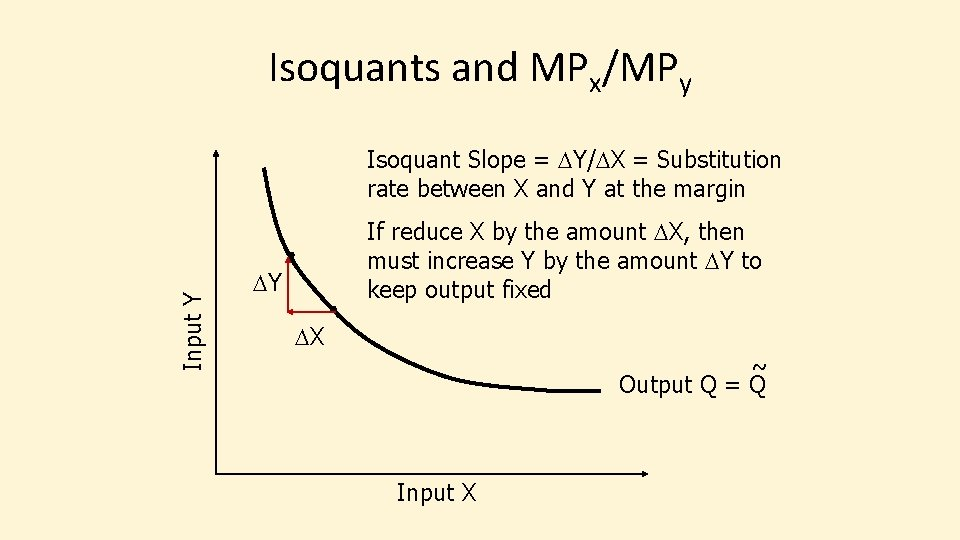Isoquants and MPx/MPy Input Y Isoquant Slope = DY/DX = Substitution rate between X and Y at the margin If reduce X by the amount DX, then must increase Y by the amount DY to keep output fixed DY DX ~ Output Q = Q Input XIsoquants and MPx/MPy Isoquant slope = d. Y/d. X = – MPx/MPy = – Ratio of MP’s Isoquant Slope = – MRTS DY Input Y d. Y/d. X = – (d. Q/d. X)/(d. Q/d. Y) DX Need minus sign since marginal products are positive and slope is negative ~ Output Q = Q Input X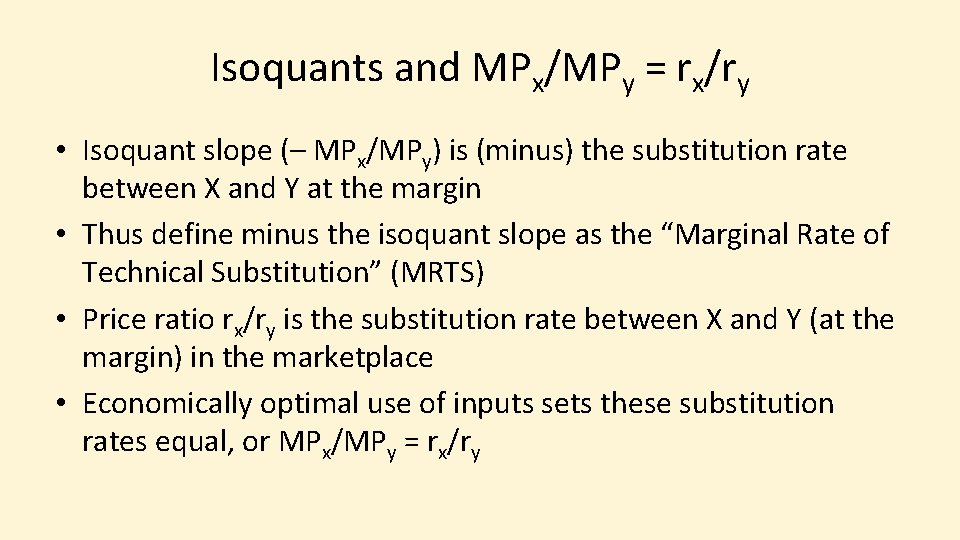Isoquants and MPx/MPy = rx/ry • Isoquant slope (– MPx/MPy) is (minus) the substitution rate between X and Y at the margin • Thus define minus the isoquant slope as the “Marginal Rate of Technical Substitution” (MRTS) • Price ratio rx/ry is the substitution rate between X and Y (at the margin) in the marketplace • Economically optimal use of inputs sets these substitution rates equal, or MPx/MPy = rx/ry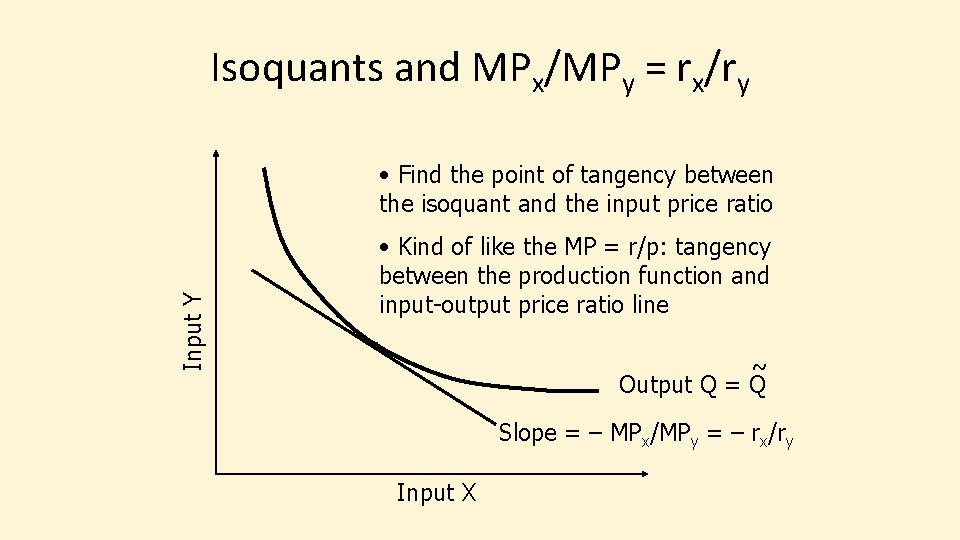Isoquants and MPx/MPy = rx/ry Input Y • Find the point of tangency between the isoquant and the input price ratio • Kind of like the MP = r/p: tangency between the production function and input-output price ratio line ~ Output Q = Q Slope = – MPx/MPy = – rx/ry Input XSoybean Meal (lbs) Corn (lbs) 10 376. 8 15 356. 3 20 339. 0 25 326. 0 30 315. 0 35 307. 5 40 300. 6 45 294. 6 50 289. 2 55 284. 4 60 280. 2 65 276. 4 70 272. 9 Soybean meal and corn needed for 125 lb feeder pigs to gain 125 lbs Which feed ration do you use?Soyb Meal Corn MRTS 10 15 20 376. 8 356. 3 339. 0 4. 10 3. 46 25 30 35 326. 0 315. 0 307. 5 2. 60 2. 20 1. 50 40 45 50 55 60 65 300. 6 294. 6 289. 2 284. 4 280. 2 276. 4 1. 38 1. 20 1. 08 0. 96 0. 84 0. 76 70 272. 9 0. 70 MRTS = As increase Soybean Meal, how much can you decrease Corn and keep output constant? • Can’t use MRTS = – MPx/MPy since DQ = 0 on an isoquant Use MRTS = – DY/DX DX = (Soy. M 2 – Soy. M 1) DY = (Corn 2 – Corn 1) = – (294. 6 – 300. 6)/(45 – 40) = – (284. 4 – 289. 2)/(55 – 50) Interpretation: If increase soybean meal 1 lb, decrease corn by 1. 20 or 0. 96 lbs and keep same gain on hogsSoy Meal (lbs) Corn (lbs) 10 376. 8 15 356. 3 4. 10 2. 20 20 339. 0 3. 46 2. 20 25 326. 0 2. 60 2. 20 30 315. 0 2. 20 35 307. 5 1. 50 2. 20 40 300. 6 1. 38 2. 20 45 294. 6 1. 20 2. 20 50 289. 2 1. 08 2. 20 55 284. 4 0. 96 2. 20 60 280. 2 0. 84 2. 20 65 276. 4 0. 76 2. 20 70 272. 9 0. 70 2. 20 MRTS P ratio 2. 20 Economically Optimal input use is where MRTS = input price ratio, or – DY/DX = rx/ry (Same as MPx/MPy = rx/ry) Soybean Meal Price \$176/ton = \$0. 088/lb Corn Price \$2. 24/bu = \$0. 04/lb Ratio: 0. 088/0. 04 = 2. 20 lbs corn/lbs soy Keep straight which is X and which is Y!!!Intuition • What does – DY/DX = rx/ry mean? • Ratio – DY/DX is how much less Y you need if you increase X, or the MRTS • Cross multiply to get – DYry = DXrx • – DYry = cost savings from decreasing Y • DXrx = cost increase from increasing X • Keep sliding down the isoquant (decreasing Y and increasing with output staying the same) as long as the cost saving exceeds the cost increase • Like a partial budget analysis of the cost changes (revenue doesn’t change, as output doesn’t change)Main Point on MRTS • When you have information on inputs needed to generate the same output, you can identify the optimal input combination • Theory: (MRTS) MPx/MPy = rx/ry • Practice: (isoquant slope) – DY/DX = rx/ry • Note how the input in the numerator and denominator changes between theory and practice • The numbers in a “table” like I have created actually have underneath them a 2 -input production function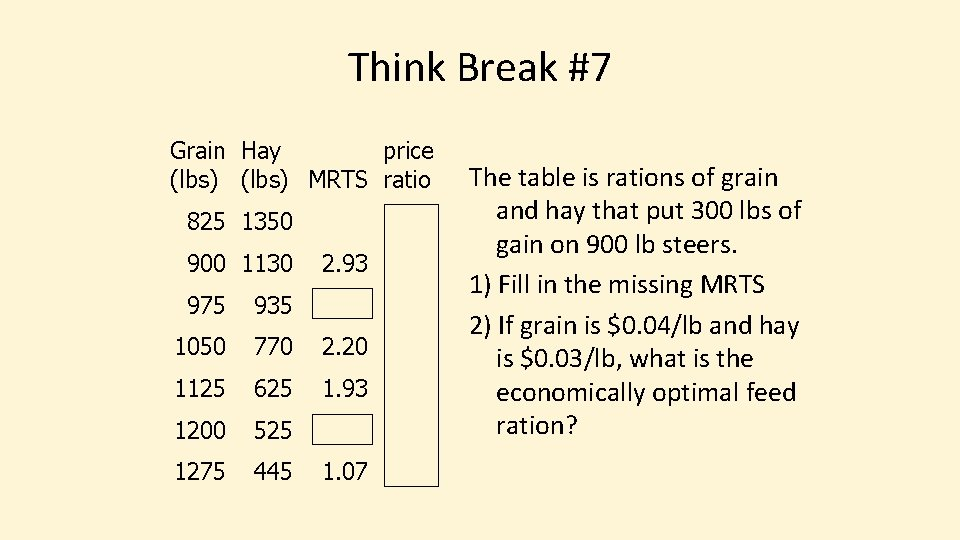Think Break #7 Grain Hay price (lbs) MRTS ratio 825 1350 900 1130 2. 93 975 935 1050 770 2. 20 1125 625 1. 93 1200 525 1275 445 1. 07 The table is rations of grain and hay that put 300 lbs of gain on 900 lb steers. 1) Fill in the missing MRTS 2) If grain is \$0. 04/lb and hay is \$0. 03/lb, what is the economically optimal feed ration?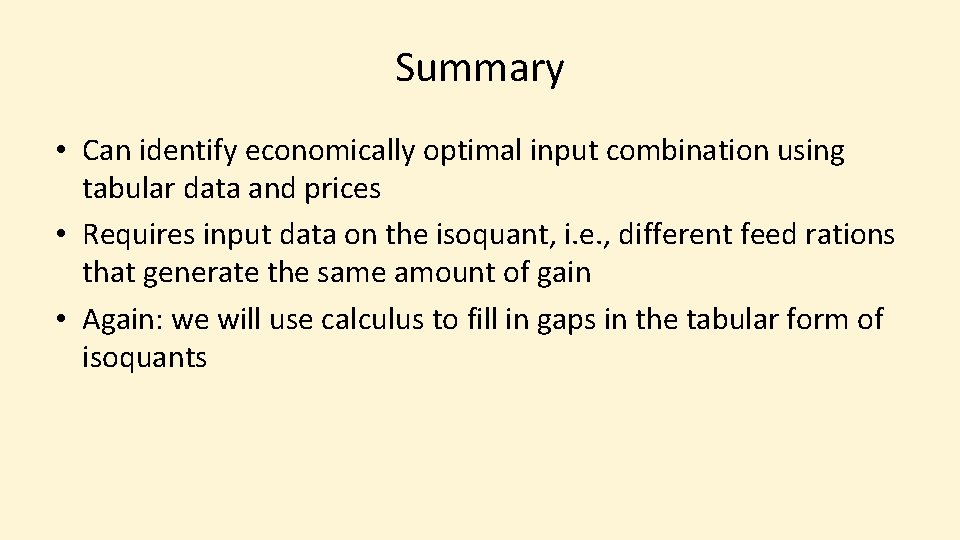Summary • Can identify economically optimal input combination using tabular data and prices • Requires input data on the isoquant, i. e. , different feed rations that generate the same amount of gain • Again: we will use calculus to fill in gaps in the tabular form of isoquants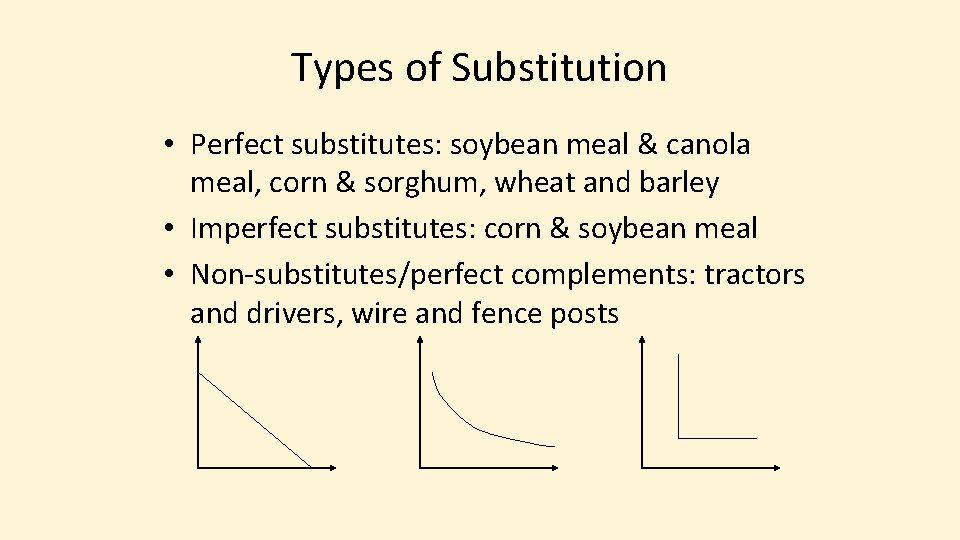Types of Substitution • Perfect substitutes: soybean meal & canola meal, corn & sorghum, wheat and barley • Imperfect substitutes: corn & soybean meal • Non-substitutes/perfect complements: tractors and drivers, wire and fence postsTypes of Input Substitution • Perfect substitutes: soybean meal & canola meal, corn & sorghum, wheat and barley • Imperfect substitutes: corn & soybean meal • Non-substitutes/perfect complements: tractors and drivers, wire and fence postsPerfect Substitutes • MRTS (slope of isoquant) is constant • Examples: canola meal and soybean meal, or corn and sorghum in a feed ration • Constant conversion between two inputs • 2 lbs of canola meal = 1 lb of soybean meal: – DCnla/DSoyb = 2 • 1. 2 bu of sorghum = 1 bu corn • – DSrgm/DCorn = 1. 2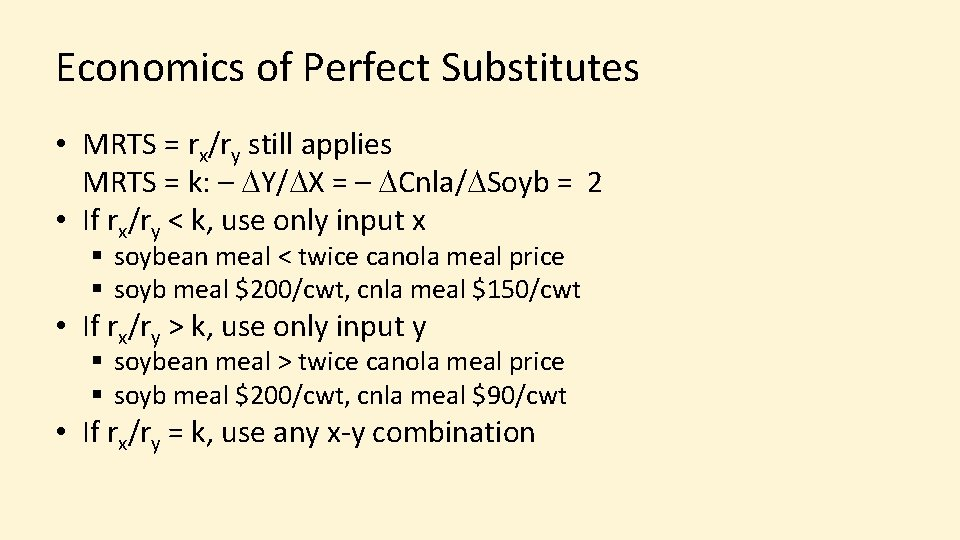Economics of Perfect Substitutes • MRTS = rx/ry still applies MRTS = k: – DY/DX = – DCnla/DSoyb = 2 • If rx/ry < k, use only input x § soybean meal < twice canola meal price § soyb meal \$200/cwt, cnla meal \$150/cwt • If rx/ry > k, use only input y § soybean meal > twice canola meal price § soyb meal \$200/cwt, cnla meal \$90/cwt • If rx/ry = k, use any x-y combination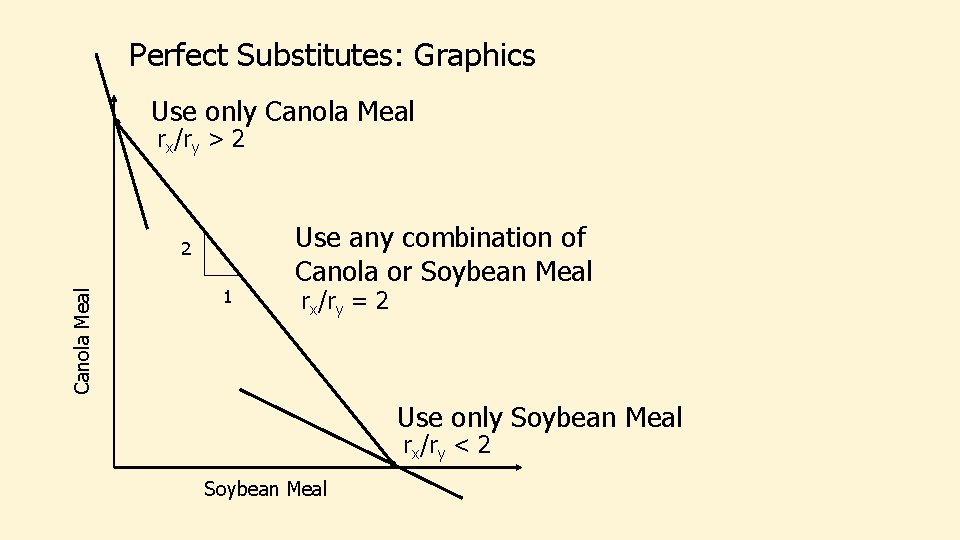Perfect Substitutes: Graphics Use only Canola Meal rx/ry > 2 Canola Meal 2 1 Use any combination of Canola or Soybean Meal rx/ry = 2 Use only Soybean Meal rx/ry < 2 Soybean MealEconomics of Imperfect Substitutes Input Y This the case we already did! Input X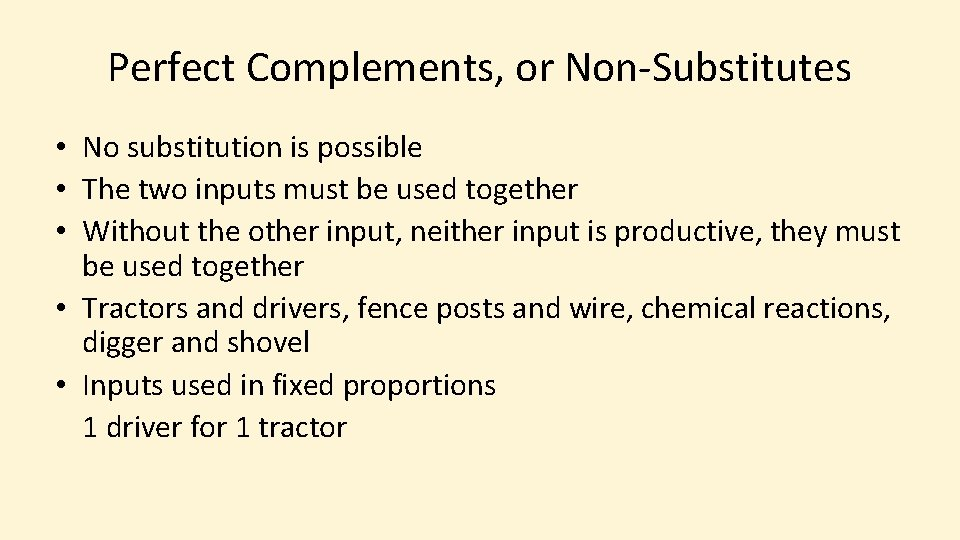Perfect Complements, or Non-Substitutes • No substitution is possible • The two inputs must be used together • Without the other input, neither input is productive, they must be used together • Tractors and drivers, fence posts and wire, chemical reactions, digger and shovel • Inputs used in fixed proportions 1 driver for 1 tractorEconomics of Perfect Complements • MRTS is undefined, the price ratio rx/ry does not identify the optimal combination • Leontief Production Function Q = min(a. X, b. Y): • Marginal products of inputs = 0 • Either an output quota or a cost budget, with the fixed input proportions defining how much of the inputs to use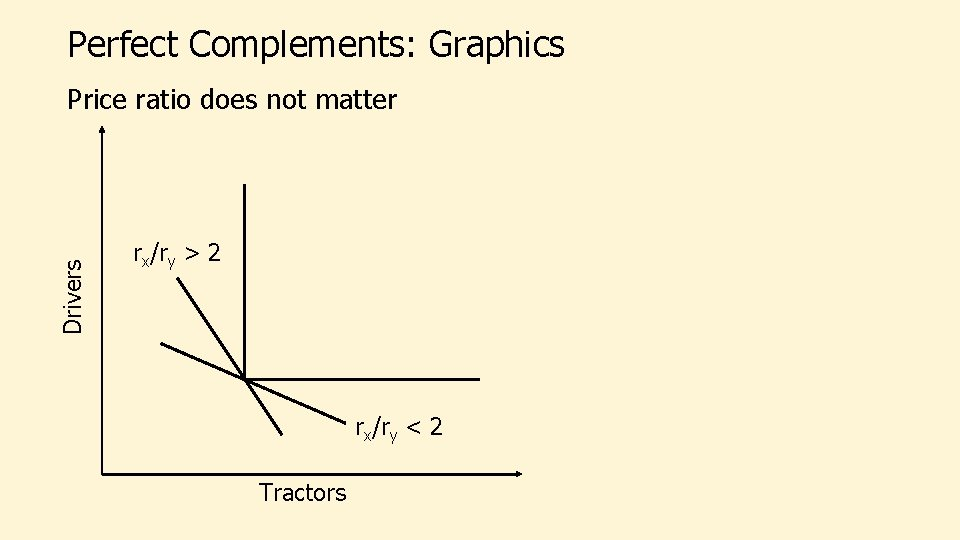Perfect Complements: Graphics Drivers Price ratio does not matter rx/ry > 2 rx/ry < 2 Tractors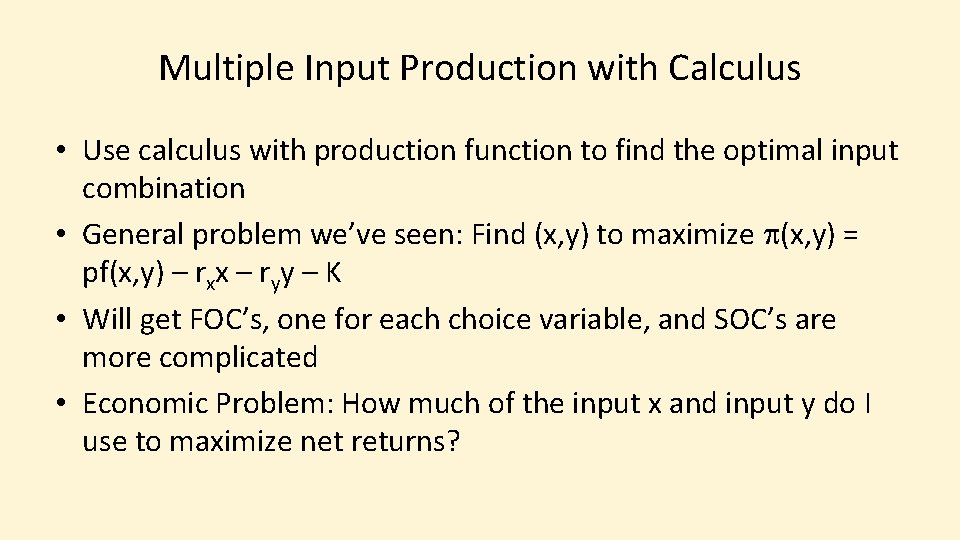Multiple Input Production with Calculus • Use calculus with production function to find the optimal input combination • General problem we’ve seen: Find (x, y) to maximize p(x, y) = pf(x, y) – rxx – ryy – K • Will get FOC’s, one for each choice variable, and SOC’s are more complicated • Economic Problem: How much of the input x and input y do I use to maximize net returns?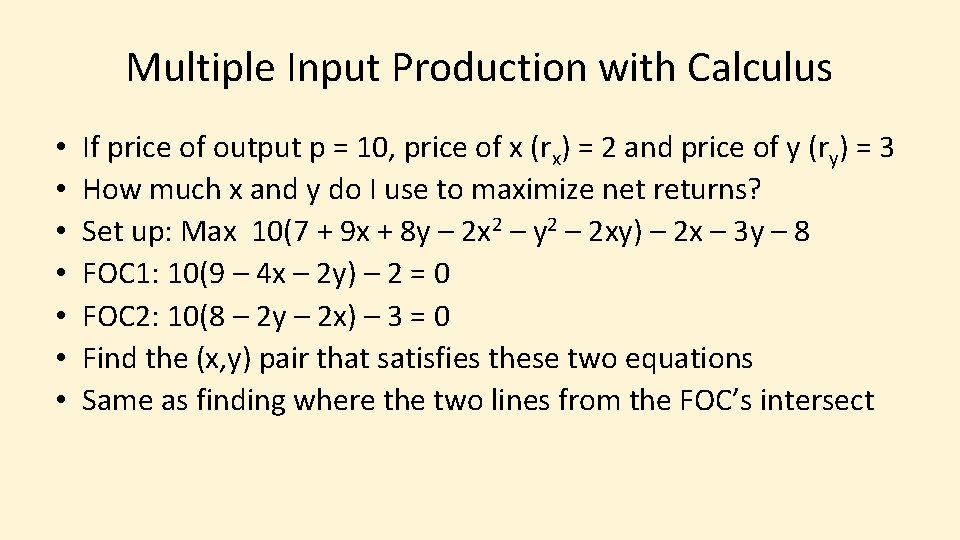Multiple Input Production with Calculus • • If price of output p = 10, price of x (rx) = 2 and price of y (ry) = 3 How much x and y do I use to maximize net returns? Set up: Max 10(7 + 9 x + 8 y – 2 x 2 – y 2 – 2 xy) – 2 x – 3 y – 8 FOC 1: 10(9 – 4 x – 2 y) – 2 = 0 FOC 2: 10(8 – 2 y – 2 x) – 3 = 0 Find the (x, y) pair that satisfies these two equations Same as finding where the two lines from the FOC’s intersect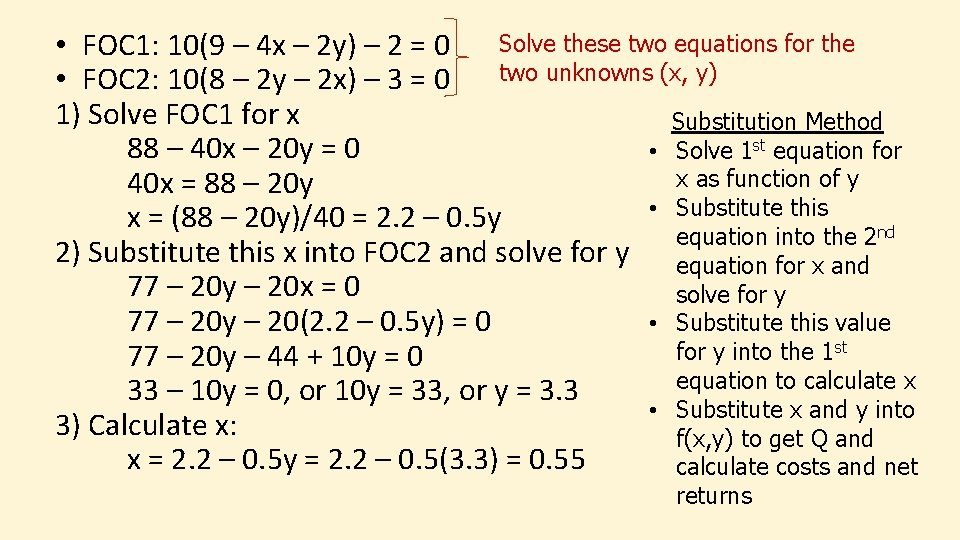Solve these two equations for the • FOC 1: 10(9 – 4 x – 2 y) – 2 = 0 two unknowns (x, y) • FOC 2: 10(8 – 2 y – 2 x) – 3 = 0 1) Solve FOC 1 for x Substitution Method 88 – 40 x – 20 y = 0 • Solve 1 st equation for x as function of y 40 x = 88 – 20 y • Substitute this x = (88 – 20 y)/40 = 2. 2 – 0. 5 y equation into the 2 nd 2) Substitute this x into FOC 2 and solve for y equation for x and 77 – 20 y – 20 x = 0 solve for y 77 – 20 y – 20(2. 2 – 0. 5 y) = 0 • Substitute this value for y into the 1 st 77 – 20 y – 44 + 10 y = 0 equation to calculate x 33 – 10 y = 0, or 10 y = 33, or y = 3. 3 • Substitute x and y into 3) Calculate x: f(x, y) to get Q and x = 2. 2 – 0. 5 y = 2. 2 – 0. 5(3. 3) = 0. 55 calculate costs and net returns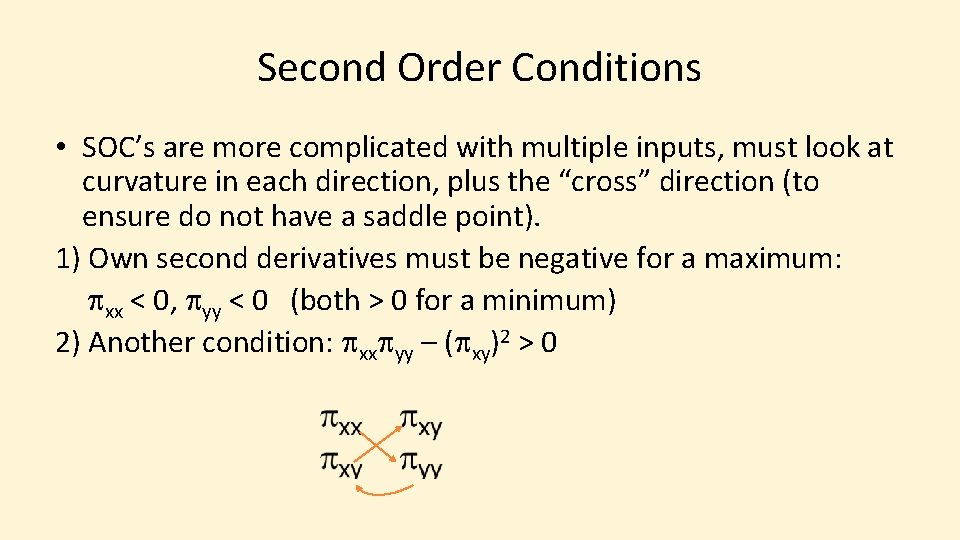Second Order Conditions • SOC’s are more complicated with multiple inputs, must look at curvature in each direction, plus the “cross” direction (to ensure do not have a saddle point). 1) Own second derivatives must be negative for a maximum: pxx < 0, pyy < 0 (both > 0 for a minimum) 2) Another condition: pxxpyy – (pxy)2 > 0• FOC 1: 10(9 – 4 x – 2 y) – 2 = 0 • FOC 2: 10(8 – 2 y – 2 x) – 3 = 0 • Second Derivatives 1) pxx: 10(– 4) = – 40 2) pyy: 10(– 2) = – 20 3) pxy: 10(– 2) = – 20 SOC’s 1) pxx = – 40 < 0 and pyy = – 20 < 0 2) pxxpyy – (pxy)2 > 0 (– 40)(– 20) – (– 20)2 = 800 – 400 > 0 Solution x = 0. 55 and y = 3. 3 is a maximumMilk Production • M = – 25. 9 + 2. 56 G + 1. 05 H – 0. 00505 G 2 – 0. 00109 H 2 – 0. 00352 GH • M = milk production (lbs/week) • G = grain (lbs/week) • H = hay (lbs/week) • Milk \$14/cwt, Grain \$3/cwt, Hay \$1. 50/cwt • What are the profit maximizing inputs? (Source: Heady and Bhide 1983)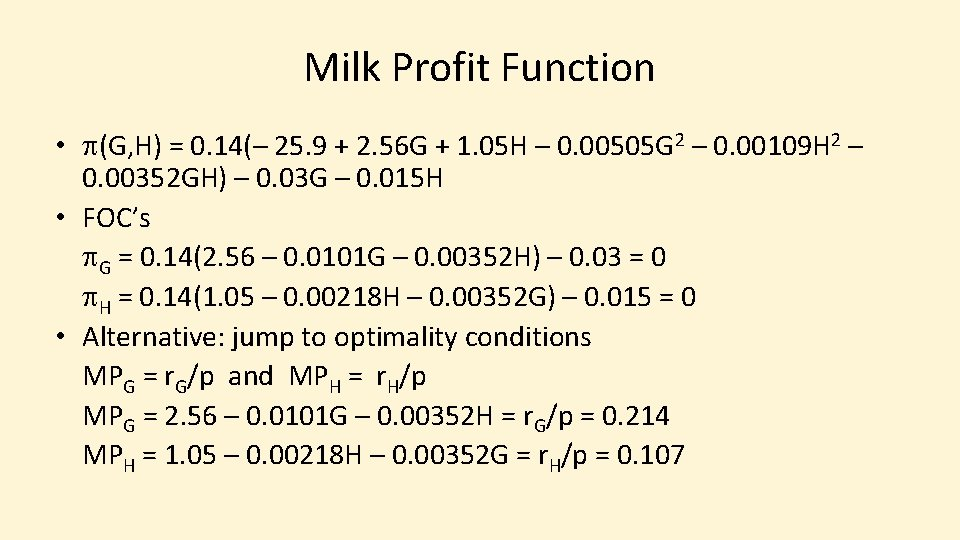Milk Profit Function • p(G, H) = 0. 14(– 25. 9 + 2. 56 G + 1. 05 H – 0. 00505 G 2 – 0. 00109 H 2 – 0. 00352 GH) – 0. 03 G – 0. 015 H • FOC’s p. G = 0. 14(2. 56 – 0. 0101 G – 0. 00352 H) – 0. 03 = 0 p. H = 0. 14(1. 05 – 0. 00218 H – 0. 00352 G) – 0. 015 = 0 • Alternative: jump to optimality conditions MPG = r. G/p and MPH = r. H/p MPG = 2. 56 – 0. 0101 G – 0. 00352 H = r. G/p = 0. 214 MPH = 1. 05 – 0. 00218 H – 0. 00352 G = r. H/p = 0. 107Solve FOC 1 for H: 2. 56 – 0. 0101 G – 0. 00352 H = 0. 214 2. 346 – 0. 0101 G = 0. 00352 H H = (2. 346 – 0. 0101 G)/0. 00352 H = 666 – 2. 87 G Substitute this H into FOC 2 and solve for G: 1. 05 – 0. 00218 H – 0. 00352 G = 0. 107 0. 943 – 0. 00218(666 – 2. 87 G) – 0. 00352 G = 0 0. 943 – 1. 45 + 0. 00626 G – 0. 00352 G = 0 0. 00274 G – 0. 507 = 0 G = 0. 507/0. 00274 = 185 lbs/week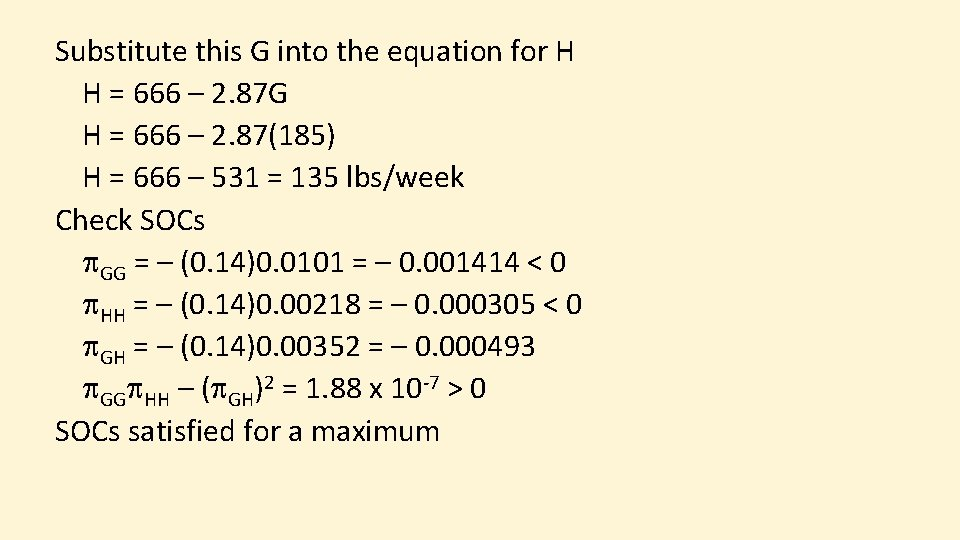Substitute this G into the equation for H H = 666 – 2. 87 G H = 666 – 2. 87(185) H = 666 – 531 = 135 lbs/week Check SOCs p. GG = – (0. 14)0. 0101 = – 0. 001414 < 0 p. HH = – (0. 14)0. 00218 = – 0. 000305 < 0 p. GH = – (0. 14)0. 00352 = – 0. 000493 p. GGp. HH – (p. GH)2 = 1. 88 x 10 -7 > 0 SOCs satisfied for a maximum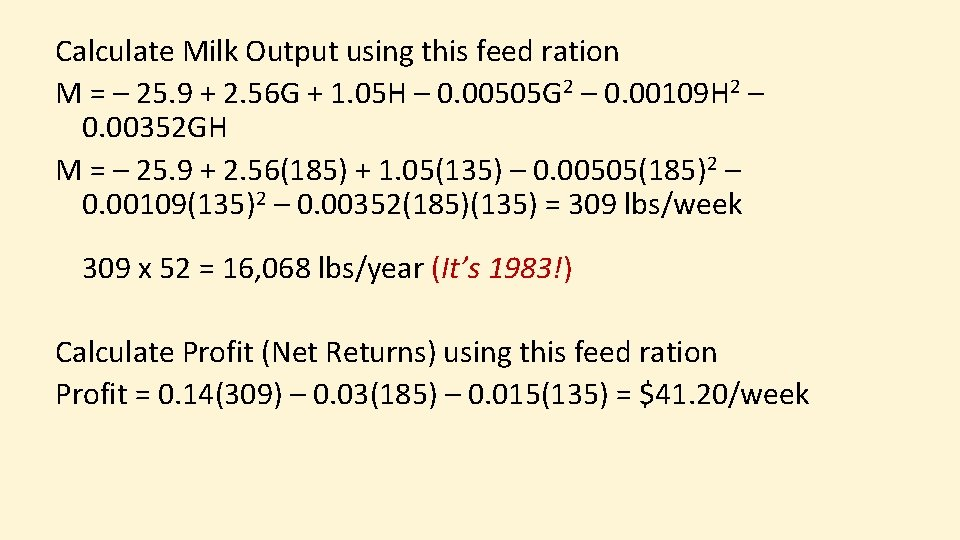Calculate Milk Output using this feed ration M = – 25. 9 + 2. 56 G + 1. 05 H – 0. 00505 G 2 – 0. 00109 H 2 – 0. 00352 GH M = – 25. 9 + 2. 56(185) + 1. 05(135) – 0. 00505(185)2 – 0. 00109(135)2 – 0. 00352(185)(135) = 309 lbs/week 309 x 52 = 16, 068 lbs/year (It’s 1983!) Calculate Profit (Net Returns) using this feed ration Profit = 0. 14(309) – 0. 03(185) – 0. 015(135) = \$41. 20/weekThink Break #8 • Repeat the previous problem, but now use a milk price of Milk \$10/cwt • M = – 25. 9 + 2. 56 G + 1. 05 H – 0. 00505 G 2 – 0. 00109 H 2 – 0. 00352 GH • Prices: Grain \$3/cwt, Hay \$1. 50/cwt • What are the profit maximizing inputs?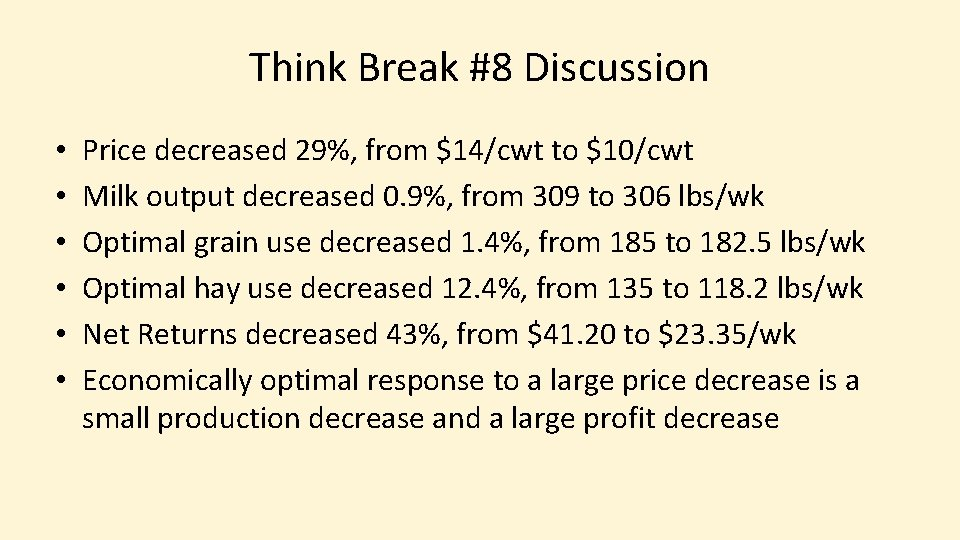Think Break #8 Discussion • • • Price decreased 29%, from \$14/cwt to \$10/cwt Milk output decreased 0. 9%, from 309 to 306 lbs/wk Optimal grain use decreased 1. 4%, from 185 to 182. 5 lbs/wk Optimal hay use decreased 12. 4%, from 135 to 118. 2 lbs/wk Net Returns decreased 43%, from \$41. 20 to \$23. 35/wk Economically optimal response to a large price decrease is a small production decrease and a large profit decrease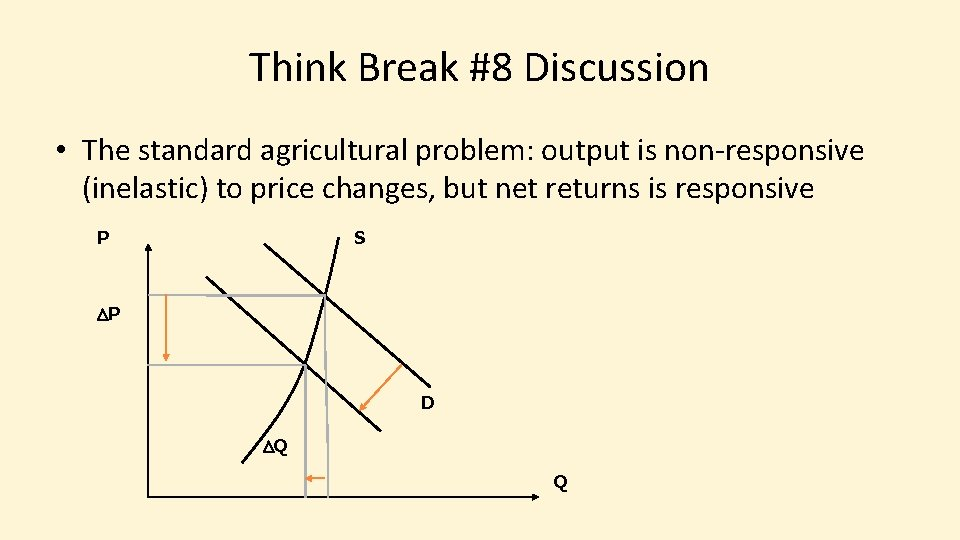Think Break #8 Discussion • The standard agricultural problem: output is non-responsive (inelastic) to price changes, but net returns is responsive P S DP D DQ QSummary • Optimality Conditions for multiple inputs have several expressions, all imply Equal Margin Principle • MRTS, isoquant, and optimal input use are all connected, know the graphics • Know the different types of input substitution • Be able to identify optimal input use • Tabular form • Using calculus